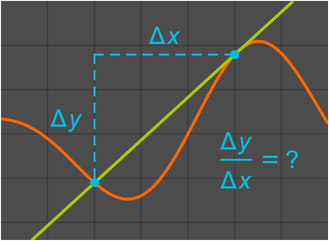# Symbolic & Numeric CalculusMathematica Version 11 builds on its capabilities in calculus, with new symbolic and numeric features that enhance each other. Integral transforms are extended with the Mellin transform, along with related convolution and function simplification operations. The symbolic differential equation solver has been extended to support multiple types of integral and integro-differential equations. A new back end has been added to the numerical optimization engine for faster and more accurate solutions for large systems. Small tweaks include a new function for computing difference quotients and extending volume computations to understand non-Cartesian coordinates. Taken together, these enhancements make calculus faster, easier, and better at all levels of sophistication.

## Key Features

 Find the Mellin transforms of functions. » Perform the Mellin convolutions for pairs of functions. » Express functions in terms of Meijer's G function. » Rapidly solve constrained numerical optimization problems with hundreds of variables. » Compute ordinary and partial difference quotients. » Obtain symbolic solutions for linear integral equations. » Solve inhomogeneous differential equations using Green's functions. » Compute areas and volumes using non-Cartesian coordinates. »